# The Envelope of The Alexander Polynomial

(diff) ← Older revision | Latest revision (diff) | Newer revision → (diff)
In Preparation

The information below is preliminary and cannot be trusted! (v)

## The Internal Kernel of the Alexander Polynomial

All that there is here comes from 06-1350/Class Notes for Tuesday October 24. Many further relevant facts are in arXiv:q-alg/9602014 by José M. Figueroa-O'Farrill, Takashi Kimura, Arkady Vaintrob and in arXiv:math.QA/0204346 by Jens Lieberum.

At the moment I know of just three relations in the internal kernel of the Alexander polynomial: the bubble relation, the H relation and the 4Y relation:

I have good reasons to suspect that there are further relations. But at the moment I don't know what they are, so below we will make do with what we have.

## Alexander-Conway, Precisely

Let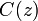$C(z)$ denote the Conway polynomial and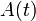$A(t)$ denote the Alexander polynomial. By we know that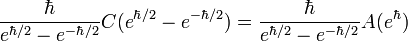$\frac{\hbar}{e^{\hbar/2}-e^{-\hbar/2}}C(e^{\hbar/2}-e^{-\hbar/2}) = \frac{\hbar}{e^{\hbar/2}-e^{-\hbar/2}}A(e^\hbar)$

is a canonical Vassiliev power series. Let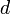$d$ denote "half a bubble". The following theorem follows easily from the above canonicity statement and the fact that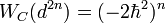$W_C(d^{2n})=(-2\hbar^2)^n$ (in shorter and less precise form,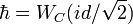$\hbar=W_C(id/\sqrt2)$), where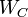$W_C$ is the weight system of the Alexander-Conway polynomial:

Theorem. Let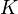$K$ be a knot and let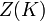$Z(K)$ be the Kontsevich integral of$K$. Then within the envelope of the Alexander-Conway polynomial,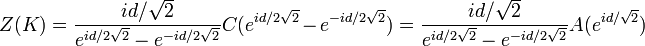$Z(K) = \frac{id/\sqrt2}{e^{id/2\sqrt2}-e^{-id/2\sqrt2}} C(e^{id/2\sqrt2}-e^{-id/2\sqrt2}) = \frac{id/\sqrt2}{e^{id/2\sqrt2}-e^{-id/2\sqrt2}} A(e^{id/\sqrt2})$.